Sonobe Star
 To the main page    "Mathematische Basteleien"

What is the Sonobe Star?
 ...... The Sonobe star is a three-dimensional star that is assembled from 30 modules.  The modules are folded from squares. Mathematically, it is an icosahedron with 20 three-sided pyramids on its sides. The name goes back to the Japanese origami enthusiast Mitsunobu Sonobe.

 ...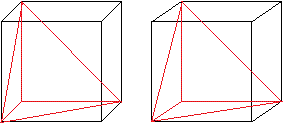... The pyramids are more exactly right-angled three-sided pyramids. ................................. They occur at one corner of a cube.  Three congruent right-angled isosceles triangles meet at the top.  The base is an equilateral triangle.

 ...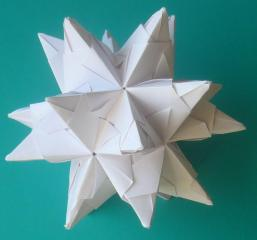... The Sonobe star is related to the Bascetta Star, which is also a stellated icosahedron and which is also assembled from 30 modules.

Folding of the Sonobe Module     top
 ...... You need 30 squares of paper for the star.  You can use the 9cm x 9cm note paper that you can buy in stationery shops, for example.

Nine steps
1
 ...... Divide the square sheet into four equal lengthwise strips by folding three times.......2
 ...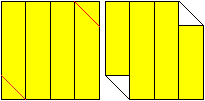... Fold on the red lines and you get the right figure................................................3
 ...... Fold on the red lines and you get the right figure.........................

4
 ...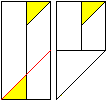... Fold on the red lines and you get the right figure.

5
 ...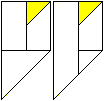... Undo the fold and tuck the lower right triangle under the left longitudinal strip......... This should look like the figure on the right.

6
 ...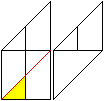Turn the sheet 180° and fold on the red line. You get the figure on the right.

7
 ...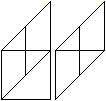Undo the fold and tuck the lower right triangle under the left longitudinal strip. You get the right figure.

8
 ...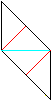...Turn the figure over. Fold on the red lines and on the blue line. Unfold again.

9
 ...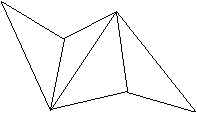This is the Sonobe module.Folding of the Sonobe Star    top
 ...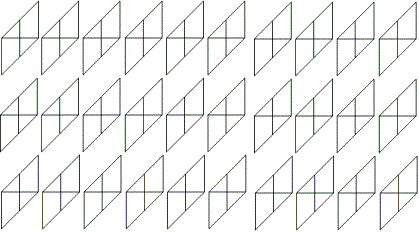... You need 30 modules for the Sonobe star. Don't worry, the module is easy to fold.

 ...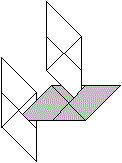...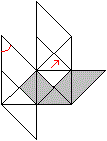First you put three modules into each other and form a pyramid corner  by pushing the tip marked in red into a pocket.

 ...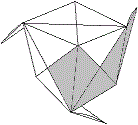... This is the view of the pyramid corner...............................................................................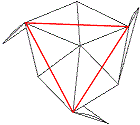... Fold along the red lines to form a right-angled pyramid with an equilateral triangle as its base.

The modules are added to the first pyramid one after the other until the star finally closes.
 ...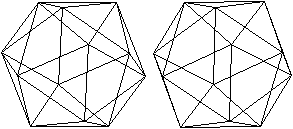... It is a great help in the resulting confusion if you imagine the generating  icosahedron: Five triangles meet at each vertex.  For the star, this means that five pyramids always form a wreath.

 ...... You can also see this in the photo of the finished star.Klasse VAB 2S der Justus-von-Liebig-Schule in Mannheim, 2015

More 3D-figures       top
Folding of an octahedron star
 ...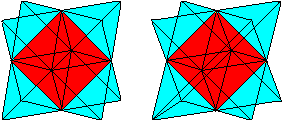... The octahedron star is created by placing tetrahedra on the eight sides of an octahedron.

 ...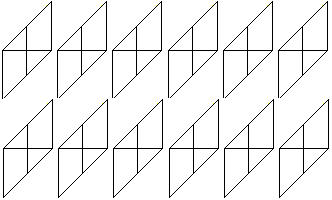... For a variant of the octahedron star you need 12 modules. The tetrahedra become right-angled three-sided pyramids.

 ...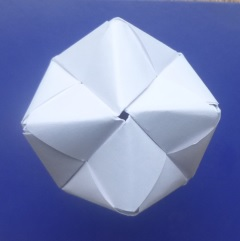... This is the star. It is put together like the Sonobe star.  You just have to make sure that not five pyramids but four pyramids form a wreath.

Folding of a Sonobe Cube
 ...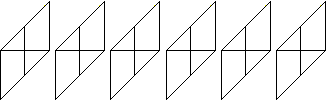... You need six modules for one cube.......First you put three modules into each other and form a cube corner,  by pushing the tip marked in red into a pocket.

 ...... This is the view of a cube corner. You add the three remaining modules one after the other and finally arrive at the Sonobe cube.

 ...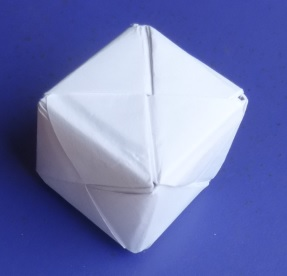...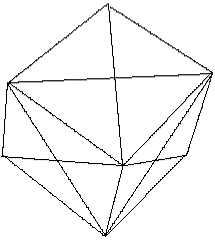Construction of a stellated cuboctahedron
 ...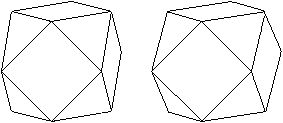... The cuboctahedron is an Archimedean solid formed by eight equilateral triangles and six squares. The Sonobe modules allow it to be stellated.

 ...... If you rebuild this figure from 24 modules, the result is disappointing. It is similar to a cube, because the square pyramids are very flat.

Playing with cubes
 ...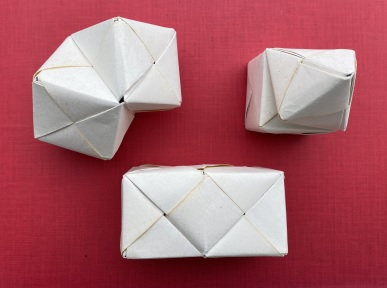Two cubes interpenetrate Cube with double pyramid piggyback Double cube

Some Mathematics       top
 ..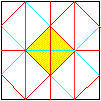. If you unfold the Sonobe module, a pattern of isosceles-rectangular triangles emerges.  The triangle coloured yellow corresponds to the side face of a right-angled pyramid.

Two questions are of interest.

Only a small part of the surface of a module is used for one pyramid. There are layers on top of each other.
What is the surface area O2 of the Sonobe star in relation to the total area O1 of the 30 initial squares?
Calculation
Let a be the length of the square side, then the side length of the basic triangle of a cone is (1/2)a.

The total area of all leaves is O1 = 30a².

The side area of a right-angled pyramid is (1/16)a².
The sum of all side areas or the surface area of the star is then O2 = 20*3*(1/16)a² = (1/4)*15a².
The ratio O2:O1 is [(1/4)*15a²]:[30a²] = 1/8 = 0.125 = 12.5 %.
Result
Only 12.5 % of the paper is used.

What is the size of the Sonobe star, if the initial square has side length a.
The size of the star should be given by the distance e of two opposite peaks.
Calculation
The distance is the sum of two heights H of the right-angled pyramids and the distance d of opposite side faces of the icosahedron, e = 2H+d.
On my website Three-Sided Pyramid you find H=(1/3)sqrt(3)*(1/2)a.
On my web page Bascetta Star you find d=(1/6)[3sqrt(3)+sqrt(15)](1/2)a.

That means e = (1/3)sqrt(3)a+(1/6)[3sqrt(3)+sqrt(15)](1/2)a = [(5/12)sqrt(3)+(1/12)sqrt(15)]a = (1/12)[5sqrt(3)+sqrt(15)]a
or rounded e = 1.044a.
Result
If a is the side length of the square sheet of paper, the Sonobe star is e = 1.044a.
If a= 9 cm, then e = 9.40 cm.

Variant of the Sonobe Modul    top
 ...... I know another variant of the Sonobe module that requires an additional folding.  The module has the advantage that it is more stable. Three modules form a bipyramid, six  a cube. - More details can be found via the two following links.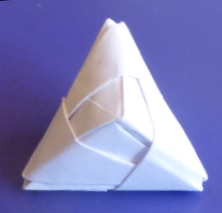Beaded Origami Ornament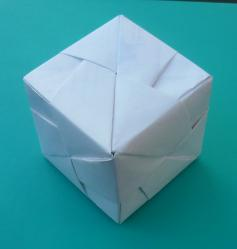Sonobe-Würfel

There is now (05/2022) another web page on a stellated icosahedron made of 20 squares, the Kusudama star.

Comparison of three stars
 a=9,0cm Sonobe Star Bascetta Star Kusudama Star 2A/Aq 12,7% 20,0% 26,8% H 0,29a = 2,6cm 0,49a = 4,4cm 0,70a = 6,3cm e 1,04a = 9,4 cm 1,45a = 13,1cm 1,97a = 17,7cm

 Sonobe Star Bascetta Star Kusudama Star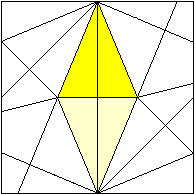Sonobe Star on the Internet    top

German

greifswalderfalterstammtisch
Sonobe-Modul   (mit Somawürfel)

English

Origami-Resource-Center.com
Sonobe Stellated Octahedron (12 units)

wikiHow
How to Make a Sonobe Unit

Wikipedia
Sonobe

Origami Modular Sonobe Unit, Sonobe instructions
 Youtube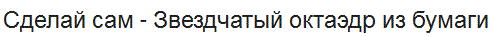DIY - Stern Oktaeder Papier   (Google Übersetzer) Even though I couldn't understand the Russian commentator, the octahedron star succeeded right away. The  pyramids are right-angled three-sided pyramids. That is why this link appears on this page. It is folded from a square sheet (cut to A4!)Feedback: Email address on my main page